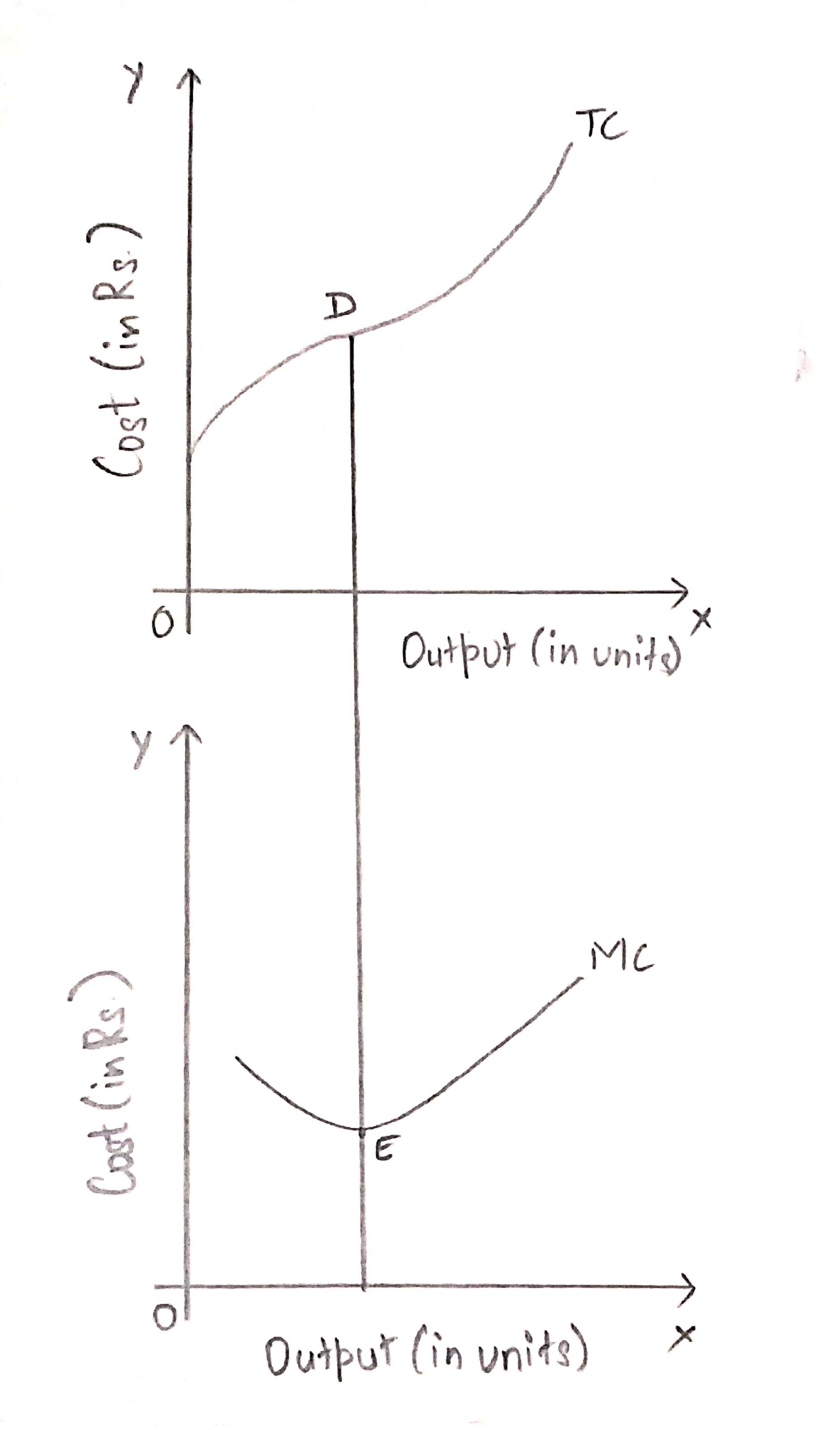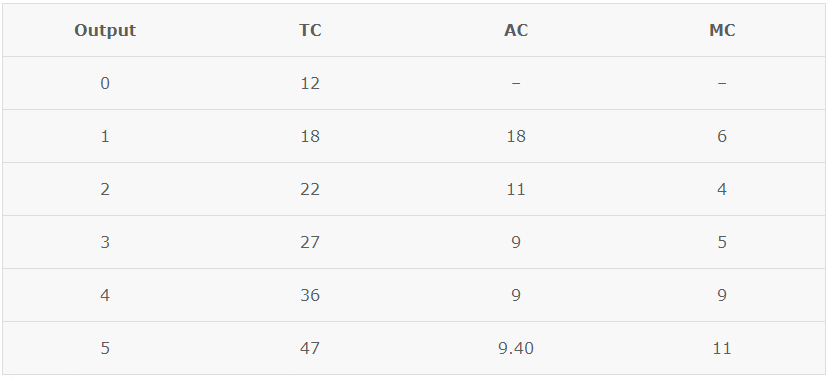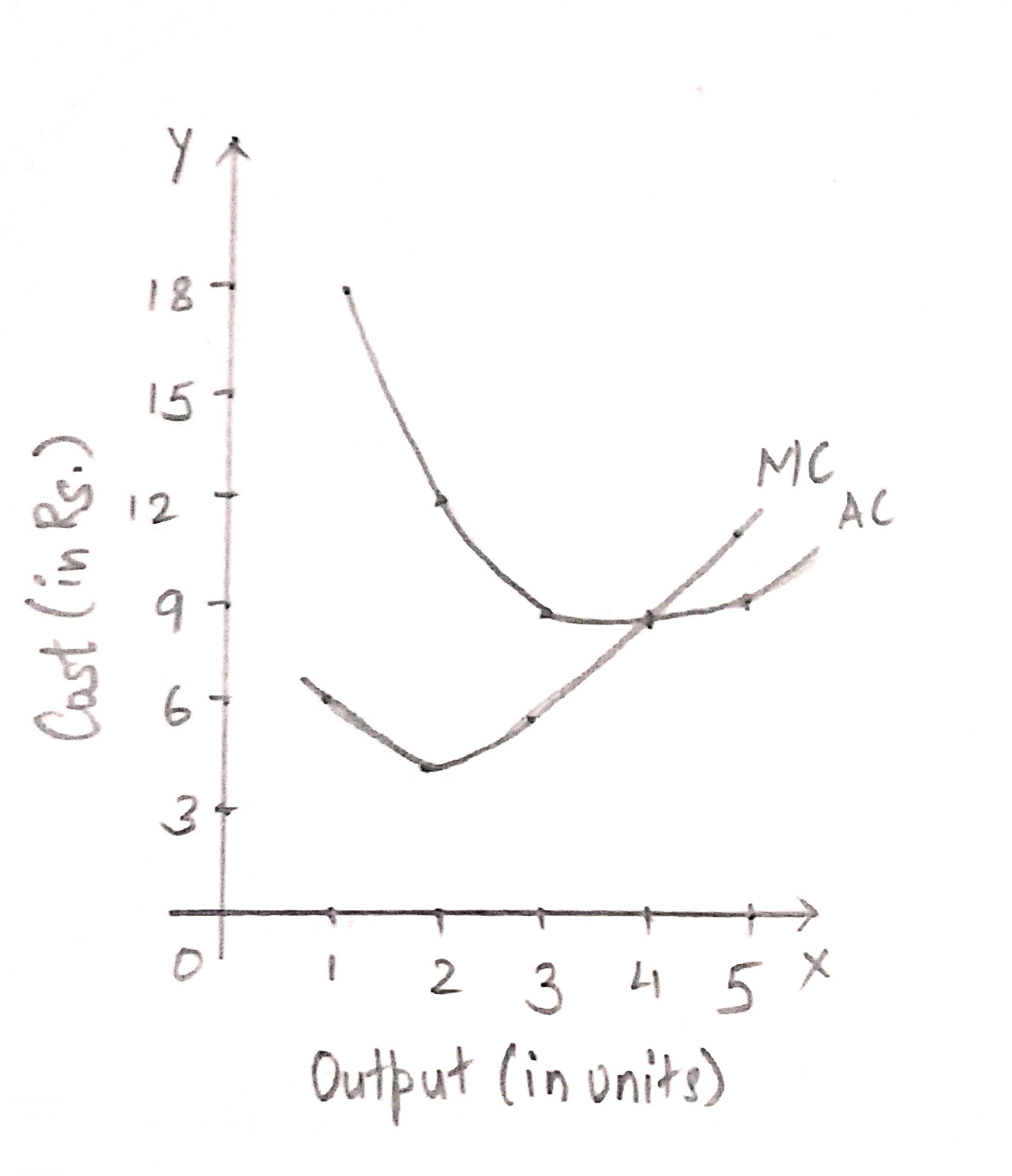# Relationship between Total Cost Marginal Cost and Average Cost

There are several types of short run costs like Average Cost (AC), Marginal Cost (MC) and Total Cost (TC). There exists a close relationship between the various types of short run costs. Let us look into the relationship between the different types of short run costs.

## Relationship between Total Cost and Marginal Cost

There is a close relationship between Total Cost and Marginal Cost. We know the marginal cost is the addition to total cost when one more unit of output is produced. When TC rises at a diminishing rate, MC declines. As the rate of increase of TC stops diminishing, MC is at its minimum point. When the rate of increase in total cost starts rising, the marginal cost increases. This concept can be better understood from the figure given below.As we can see from the graph given above, when TC rises at a diminishing rate till point D, MC declines. MC reaches its minimum point at point E. When the rate of increase of TC starts rising after point d, MC also starts increasing after point E.

## Relationship between Average Cost and Marginal Cost

Both AC and MC are derived from TC. AC refers to TC per unit output and MC refers to addition to TC when one more unit of output is produced. Also, both MC and AC curves are U-shaped due to the Law of Variable Proportions. The relationship between Average Cost and Marginal Cost can be better illustrated through the following schedule and diagram,When MC is less than AC, AC falls with increase in output, i.e. till 3 units of output. AS MC becomes equal to AC, i.e. when MC curve intersects the AC curve, AC is constant and at its minimum point. When MC is more than AC, AC rises with increase in output, i.e. from 5 units of output. Thereafter, both AC and MC rise, but MC increases at a faster rate as compared to AC. As a result MC curve us steeper than the AC curve.